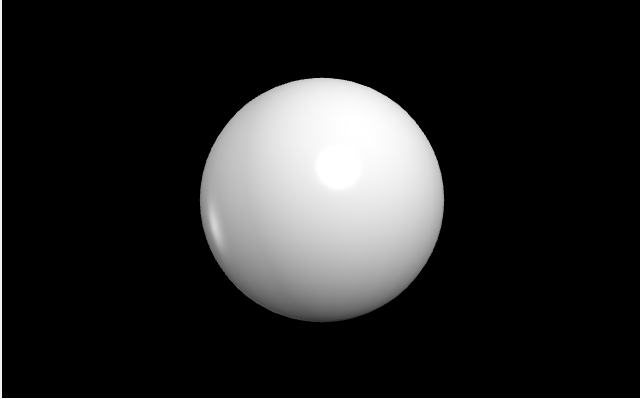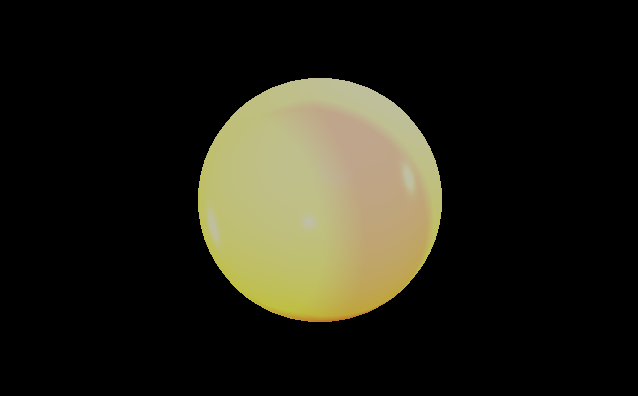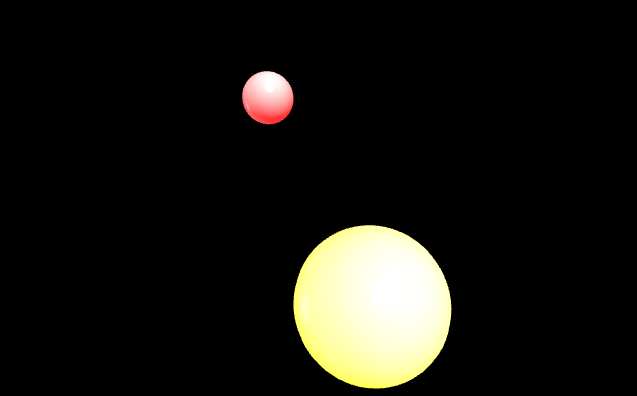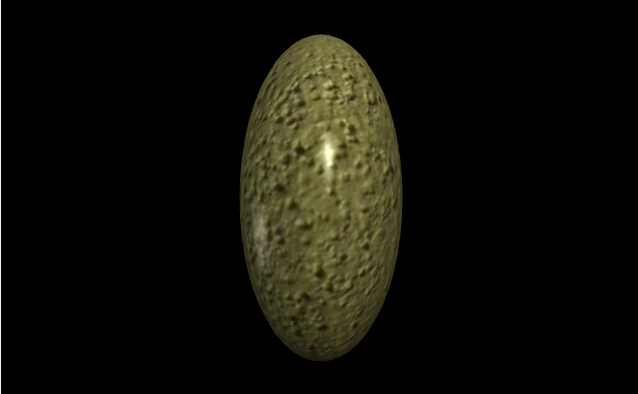GeeksforGeeks App
Open AppBrowser
Continue

# Making a sphere with VPython

`VPython` makes it easy to create navigable 3D displays and animations, even for those with limited programming experience. Because it is based on Python, it also has much to offer for experienced programmers and researchers. `VPython` allows users to create objects such as spheres and cones in 3D space and displays these objects in a window. This makes it easy to create simple visualizations, allowing programmers to focus more on the computational aspect of their programs. The simplicity of `VPython` has made it a tool for the illustration of simple physics, especially in the educational environment.

Installation :

`pip install vpython`

A sphere is a geometrical object in three-dimensional space that is the surface of a ball. We can generate a sphere in `VPython` using the `sphere()` method.

## sphere()

Syntax : sphere(parameters)

Parameters :

• pos : It is the position of the center of the sphere. Assign a vector containing 3 values, example pos = vector(0, 0, 0)
• axis : It is the axis of alignment of the sphere. Assign a vector containing 3 values, example axis = vector(1, 2, 1)
• up : It is the orientation of the sphere. Assign a vector containing 3 values, example up = vector(0, 1, 0)
• color : It is the color of the sphere. Assign a vector containing 3 values, example color = vector(1, 1, 1) will give the color white
• opacity : It is the opacity of the sphere. Assign a floating value in which 1 is the most opaque and 0 the least opaque, example opacity = 0.5
• shininess : It is the shininess of the sphere. Assign a floating value in which 1 is the most shiny and 0 the least shiny, example shininess = 0.6
• emissive : It is the emissivity of the sphere. Assign a boolean value in which True is emissive and False is not emissive, example emissivity = False
• texture : It is the texture of the sphere. Assign the required texture from the textures class, example texture = textures.stucco
• radius : It is the radius of the sphere. Assign a floating value, the default radius is 1, example radius = 10
• size : It is the size of the sphere. Assign a vector containing 3 values representing the length, height and weight respectively, the default size is (2, 2, 2), example size = vector(1, 1, 1)

All the parameters are optional.

Example 1 :A sphere with no parameters, all the parameters will have the default value.

 `# import the module``from` `vpython ``import` `*` `sphere()`

Output :Example 2 :A sphere using the parameters color, opacity, shininess and emissivity.

 `# import the module``from` `vpython ``import` `*` `sphere(color ``=` `vector(``5``, ``3``, ``1``), ``       ``opacity ``=` `0.5``, ``       ``shininess ``=` `1``, ``       ``emissive ``=` `False``)`

Output :Example 3 :Displaying 2 spheres to visualize the attributes pos and size.

 `# import the module``from` `vpython ``import` `*`` ` `# the first sphere``sphere(pos ``=` `vector(``-``1``, ``2``, ``0``),``       ``size ``=` `vector(``1``, ``1``, ``1``),``       ``color ``=` `vector(``15``, ``1``, ``1``))`` ` `# the second sphere``sphere(pos ``=` `vector(``1``, ``-``2``, ``0``), ``       ``size ``=` `vector(``3``, ``3``, ``3``),``       ``color ``=` `vector(``5``, ``10``, ``1``))`

Output :Example 4 :A sphere using the parameters texture, axis and up.

 `# import the module``from` `vpython ``import` `*` `sphere(texture ``=` `textures.stucco,``       ``axis ``=` `vector(``1``, ``0``, ``0``),``       ``up ``=` `vector(``0``, ``1``, ``0``))`

Output :
<img src="https://media.geeksforgeeks.org/wp-content/uploads/20200528102735/Screenshot-My Personal Notes arrow_drop_up